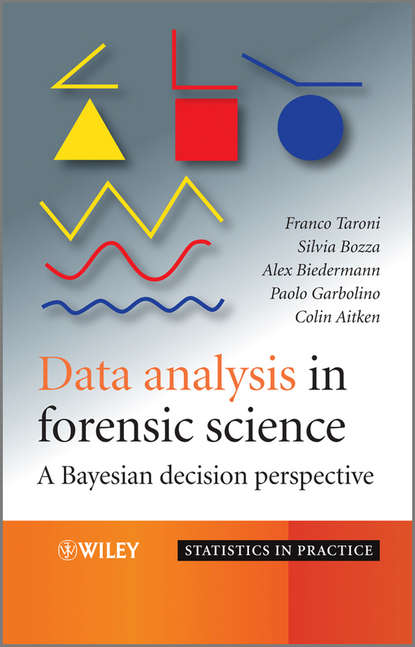Data Analysis in Forensic Science. A Bayesian Decision Perspective

# Data Analysis in Forensic Science. A Bayesian Decision Perspective

Автор
Год написания книги
2018
This is the first text to examine the use of statistical methods in forensic science and bayesian statistics in combination. The book is split into two parts: Part One concentrates on the philosophies of statistical inference. Chapter One examines the differences between the frequentist, the likelihood and the Bayesian perspectives, before Chapter Two explores the Bayesian decision-theoretic perspective further, and looks at the benefits it carries. Part Two then introduces the reader to the practical aspects involved: the application, interpretation, summary and presentation of data analyses are all examined from a Bayesian decision-theoretic perspective. A wide range of statistical methods, essential in the analysis of forensic scientific data is explored. These include the comparison of allele proportions in populations, the comparison of means, the choice of sampling size, and the discrimination of items of evidence of unknown origin into predefined populations. Throughout this practical appraisal there are a wide variety of examples taken from the routine work of forensic scientists. These applications are demonstrated in the ever-more popular R language. The reader is taken through these applied examples in a step-by-step approach, discussing the methods at each stage.

## Читать онлайн

Авторизуйтесь чтобы можно было оставлять комментарии

## Отзывы

список сообщений пуст